# Induced fibre bundle

(diff) ← Older revision | Latest revision (diff) | Newer revision → (diff)

induced fibration

The fibration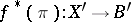induced by the mappingand the fibration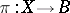, where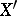is the subspace of the direct productconsisting of the pairs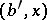for which, and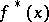is the mapping defined by the projection. The mappingfrom the induced fibre bundle into the original fibre bundle defined by the formula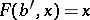is a bundle morphism covering. For each point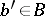, the restrictions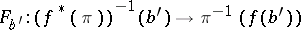are homeomorphisms. Furthermore, for any fibrationand morphismcoveringthere exist precisely one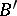-morphism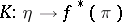such that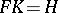,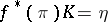and such that the following diagram is commutative: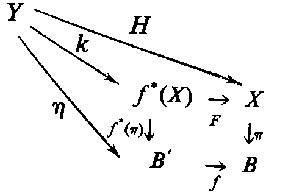Figure: i050720a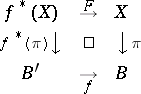Fibre bundles induced from isomorphic fibrations are isomorphic, a fibre bundle induced by a constant mapping is isomorphic to the trivial fibre bundle.

For any section of a fibration, the mapping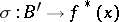defined by the formulais a section of the induced fibration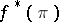and satisfies the relation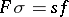. For example, the mappinginduces the fibration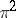with space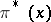and basethat is the square of the fibrationand has the canonical section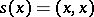.

How to Cite This Entry:
Induced fibre bundle. Encyclopedia of Mathematics. URL: http://encyclopediaofmath.org/index.php?title=Induced_fibre_bundle&oldid=12456
This article was adapted from an original article by M.I. Voitsekhovskii (originator), which appeared in Encyclopedia of Mathematics - ISBN 1402006098. See original article